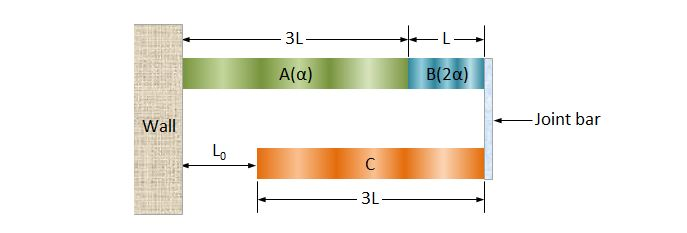# Triple metalsTwo metal sticks A and B are fixed on the left wall in the above diagram. The lengths and the linear expansion coefficients of A and B are $3L$, $\alpha$ and $L$,$2\alpha$, respectively. A third metal stick C with length $3L$ is fixed on the right joint bar on which the right end of B is also fixed. Suppose that there is a temperature change $\Delta T$ which is the same for all of A, B and C, and the distance between the wall and C remains constant, which is $L_0$ as shown in the diagram. What is the linear expansion coefficients of the metal C?

×